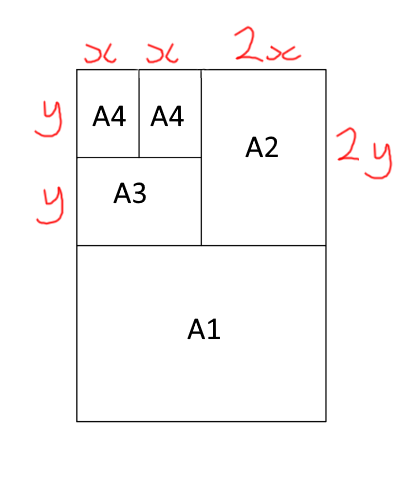#### You may also like### Matter of Scale

Can you prove Pythagoras' Theorem using enlargements and scale factors?### Conical Bottle

A right circular cone is filled with liquid to a depth of half its vertical height. The cone is inverted. How high up the vertical height of the cone will the liquid rise?### Squirty

Using a ruler, pencil and compasses only, it is possible to construct a square inside any triangle so that all four vertices touch the sides of the triangle.

# Fit for Photocopying

##### Age 14 to 16Challenge LevelYou may be familiar with the standard paper size A4.

Two sheets of A4 fit together to make a sheet of A3, two sheets of A3 fit together to make a sheet of A2, and so on.

Each member of the A paper size family is an enlargement of the others - they are all similar shapes.

Can you work out the ratio of the shorter to the longer side of a piece of A paper?
If you're not sure where to start, click below for a hint.

Alison labelled the shorter side of her A4 paper $x$, and the longer side $y$:As the rectangles are similar, she knew that $x:y = 2y:4x$.
Can you use this to work out an expression for $y$ in terms of $x$?

A sheet of A0 has an area of 1 square metre.
Can you use this fact to deduce the length and width of the different A paper sizes?

On a photocopier, approximately what percentage would you need to scale by in order to photocopy an A3 poster onto A4 paper?

Here are some challenging questions to consider:

Can you find a consistent way to define A(-1) and other negative paper sizes?

Can you find a consistent way to define A$(\frac{1}{2})$, and other fractional paper sizes?